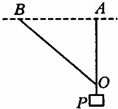# Statics with Trigonometry!As shown in the figure, the non-stretch light rope $AO$ and $BO$ are attached to a node $O$, $O$ is attached to an object $P$, $BO>AO$, where point $A$ and $B$ are fixed on the same horizontal line. At the beginning, $AO$ is vertical and the two ropes are just stretched. The tensions of $AO$ and $BO$ are denoted as $F_{A}$ and $F_{B}$ respectively. Now, keeping $A$ and $B$ on the same horizontal line, if we slowly move point $B$ to the left, what can we say about $F_{A}$ and $F_{B}$ as $AB$ increases?

Note: Can you rigorously prove it?

×